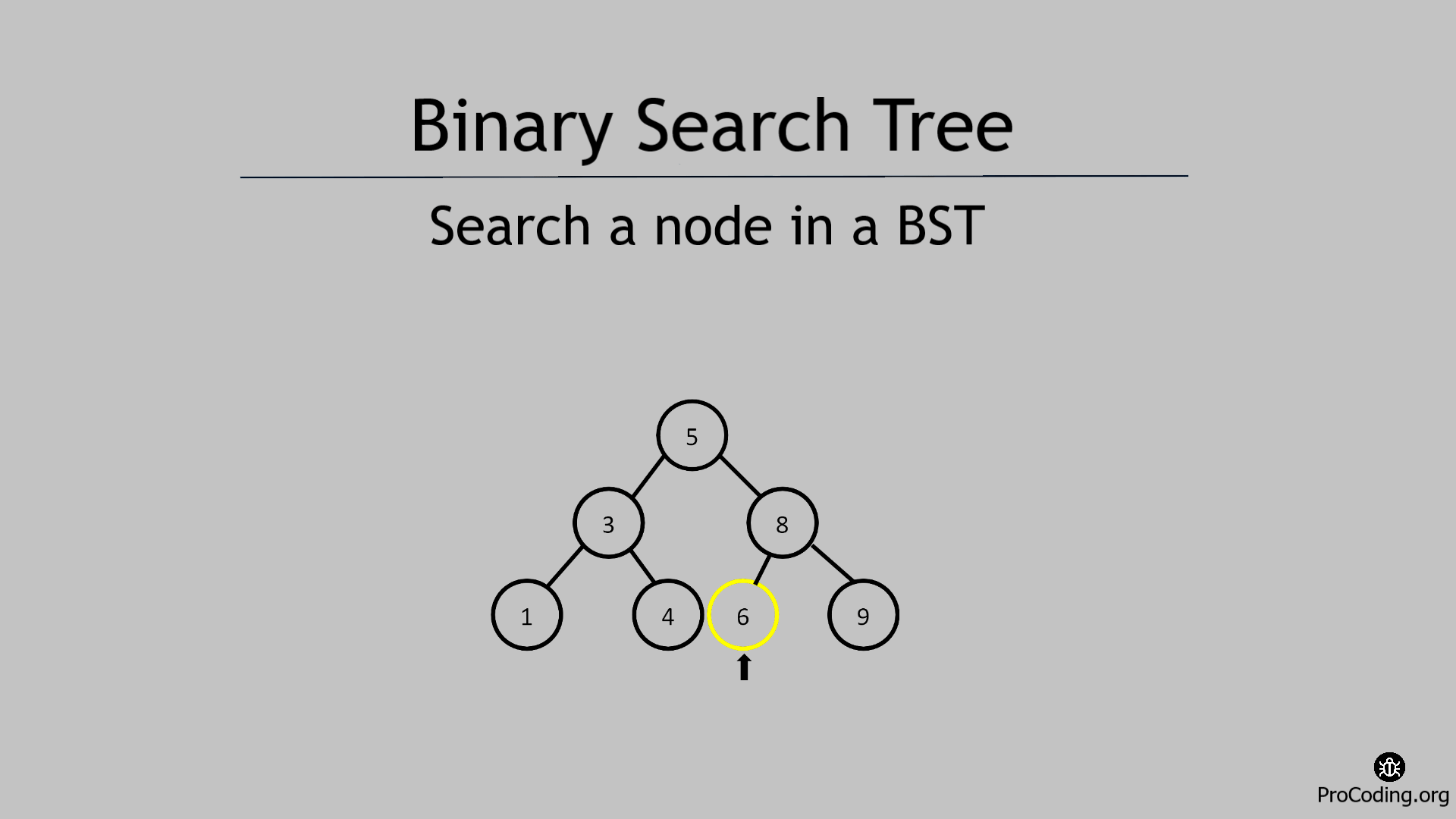# Search a node in Binary search tree

Abhishek Kumar Category: Data StructuresTags: datastructures tree binary-search-tree

In this tutorial, we are going to learn that how to search a node in a Binary search tree using recursive and iterative solution.While searching a node in a Binary Tree , we traverse each node one by one and check if required node is available or not.

But in case of Binary Search Tree, we are not required to traverse and check each and every node. Here we can compare the root node and if it is equal to the root node then search is complete otherwise check if node to be searched is less than or greater than the root node.

If the node to be searched is less than root node then ignore the right subtree and continue with the same approach in left subtree or if that node is greater than root node then ignore the left subtree and continue with the same approach in right subtree.

If the node to be searched is available in the BST then return `True` otherwise return `False`.

##### Example -
```Input:
5
/   \
3     8
/ \   / \
1   4 6   9

Node to be searched: 6

Output: True```

We can solve this problem in two ways-

### Recursive solution

###### Algorithm
Find_Recur(root, data)
``````1.  if root is null then return False
2.  if root.data == data then return True
3.  else
4.    if data < root.data
5.      return Find_Recur(root.left, data)
6.    else
7.      return Find_Recur(root.right, data)``````
``````class Node:
def __init__(self, data):
self.left = None
self.data = data
self.right = None

def find(root, data):
if not root: return False
if root.data == data: return True
else:
if data < root.data:
return find(root.left, data)
else:
return find(root.right, data)

bst_root = Node(10)
bst_root.left = Node(6)
bst_root.right = Node(16)
bst_root.left.left = Node(4)
bst_root.left.right = Node(9)
bst_root.right.left = Node(13)
bst_root.right.right = Node(20)
print(find(bst_root, 16))``````

Output

`True`

### Iterative solution

###### Algorithm
Find_Iter(root, data)
``````1.  node = root
2.  while node is not null
3.    if node.data == data then
4.      return True
5.    if data < node.data
6.      node = node.left
7.    else
8.      node = node.right
8.  return False``````
``````class Node:
def __init__(self, data):
self.left = None
self.data = data
self.right = None

def find(root, data):
node = root
while node:
if node.data == data: return True
if data < node.data:
node = node.left
else:
node = node.right
return False

bst_root = Node(10)
bst_root.left = Node(6)
bst_root.right = Node(16)
bst_root.left.left = Node(4)
bst_root.left.right = Node(9)
bst_root.right.left = Node(13)
bst_root.right.right = Node(20)
print(find(bst_root, 16))``````

#### Output

Output

`True`

Time complexity: The time complexity of both of these solution will be O(log n) if the tree is balanced but in worst case the time complete will be O(n) if the tree is not balanced i.e, tree becomes skewed.

AUTHOR### Abhishek Kumar

Software engineer | Blogger | Keen learner
Python | Django | JavaScript | React | Next.js | Express.js | C
Passionate about learning and experimenting with new things and open to more opportunities and collaborations.